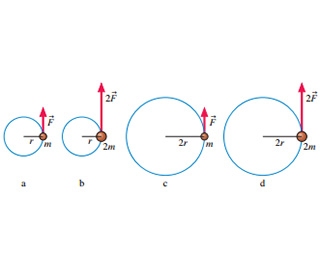# Problem: Rank in order, from largest to smallest, the angular accelerations a to d in .

###### FREE Expert Solution

Angular acceleration is given by:

$\overline{){\mathbf{\alpha }}{\mathbf{=}}\frac{\mathbf{\tau }}{\mathbf{I}}}$, where τ is torque and I is the moment of inertia.

Torque is expressed as:

$\overline{){\mathbf{\tau }}{\mathbf{=}}{\mathbf{r}}{\mathbf{F}}}$, r is radius and F is force.

Rotational inertia of an object is:

$\overline{){\mathbf{I}}{\mathbf{=}}{\mathbf{m}}{{\mathbf{r}}}^{{\mathbf{2}}}}$, where m is mass.

Therefore, angular acceleration is given by:###### Problem Details

Rank in order, from largest to smallest, the angular accelerations a to d in.